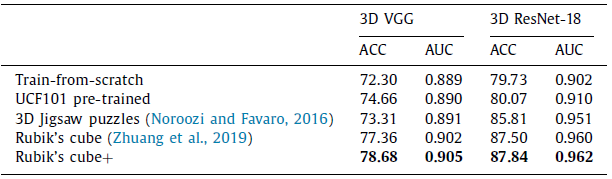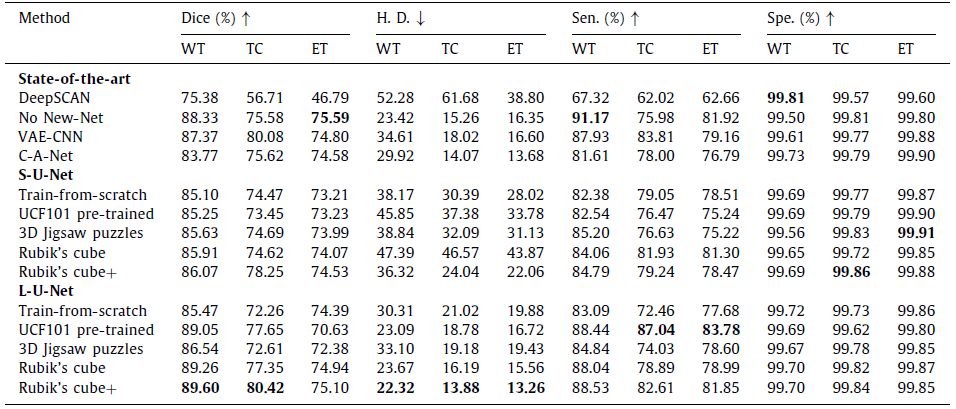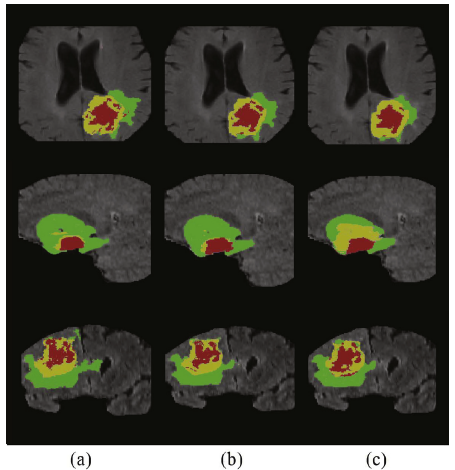# Brief Review — Rubik’s Cube+: A self-supervised feature learning framework for 3D medical image analysis

## Self-Supervised Learning by Playing Rubik’s Cube

Rubik’s Cube+: A self-supervised feature learning framework for 3D medical image analysis,
Rubik’s Cube+
, by Chinese Academy of Sciences, and Tencent Jarvis Lab,
2020 JMIA, Over 50 Citations (Sik-Ho Tsang @ Medium)
Self-Supervised Learning, Medical Image Segmentation, Medical Image Classification, Image Segmentation, Image Classification

• A pretext task, Rubik’s Cube+, is proposed, which involves three operations, namely cube ordering, cube rotating and cube masking.
• Compared to Rubik’s Cube, cube masking is added for the pretext task.

# Outline

1. Rubik’s Cube+
2. Results

# 1. Rubik’s Cube+

## 1.1. Network Architecture

• A 3D medical volume is first partitioned it into a grid (e.g., 2×2×2) of cubes, and then permuted with random rotations, as well as masking.
• The cube intensities are normalized to [−1, 1].
• A Siamese network with M (which is the number of cubes) sharing weight branches, namely Siamese-Octad, is adopted to solve Rubik’s cube.
• 3D CNN, e.g., 3D ResNet & 3D VGG are used.
• The feature maps from the last fully-connected or convolution layer of all branches are concatenated and given as input to the fully-connected layer of separate tasks, i.e., cube ordering and orientating, which are supervised by permutation loss (LP) and rotation loss (LR), respectively.

## 1.2. Permutation Loss

• Same as the one in Rubik’s Cube, the network needs to predict the permutation from the K options, which can be seen as a classification task with K categories. The corresponding loss is:

## 1.3. Rotation Loss

• Same as the one in Rubik’s Cube. The directions for cube rotation are limited, i.e., only allowing 180◦ horizontal and vertical rotations. The network is required to recognize whether each of the input cubes has been rotated. The predictions of this task are two 1×M vectors (r) indicating the possibilities of horizontal (hor) and vertical (ver) rotations for each cube. The corresponding loss is:

## 1.4. Masking Loss

• The process of random masking strategy can be summarized as:
1. First, a random possibility (pos ∈ [0, 1]) is generated for each cube to determine whether it should be masked or not.
2. If the cube is to be masked (i.e., pos≥0.5), we generate a 3D matrix R of the same shape of a cube to randomly block the content. The value of each voxel of R is a probability (prob) randomly captured from a uniform distribution [0, 1].
3. To obtain the mask, a thresholding operation ( th_R=0.5 ) is performed to R, which leads the voxel value of (x, y, z) to be 1 for prob(x, y, z)≥th_R and 0 versa vice.
• The mask identification as a multi-label classification task, which is similar to cube orientation. Let gb represent a 1 ×M ground truth for cube masking, where 1 on the position of masked cubes and 0 vice versa, and b is the M prediction.
• Then, the masking identification loss (LM) can be defined as:

## 1.5. Full Objectives

• The total loss is the sum of LP, LR & LM:
• where the loss weighting ratio is 1:1:1 indeed.

# 2. Results

## 2.1. Target Task: ClassificationThe average classification accuracy (ACC %) and the area under curve (AUC) of 3D networks trained with different strategies on the validation set of cerebral hemorrhage dataset
• Due to the gap between natural video and volumetric medical data, the improvement yielded by UCF101 pre-trained weights is limited.

The Rubik’s cube+ pre-trained 3D VGG and ResNet-18 achieve ACCs of 78.68% and 87.84%, which are 6.38% and 8.11% higher than the train-from-scratch networks, respectively.

## 2.2. Target Task: SegmentationThe segmentation accuracy of different approaches on the BraTS-2018 validation set

The Rubik’s cube+ pre-trained L-U-Net achieves the relatively high Dice for whole tumor (89.60%), tumor core (80.42%) and enhancing tumor (75.10%), which are +4.13% , +8.16% and +0.71% higher than the train-from-scratch.Visualization of brain tumor segmentation results
• Green, yellow and red stand for the whole tumor, tumor core and enhancing tumor
• Compared to train-from-scratch, the L-U-Net pre-trained on Rubik’s cube+ pretext task produces more plausible segmentation results, especially for the tumor core and enhancing tumor.

## Reference

[2020 JMIA] [Rubik’s Cube+]
Rubik’s Cube+: A self-supervised feature learning framework for 3D medical image analysis

## Self-Supervised Learning

19932020 … [Rubik’s Cube+] 2021 [MoCo v3] [SimSiam] [DINO] [Exemplar-v1, Exemplar-v2] [MICLe] [Barlow Twins]

## Biomedical Image Classification

2019 [CheXpert] [Rubik’s Cube] 2020 [VGGNet for COVID-19] [Dermatology] [Rubik’s Cube+] 2021 [MICLe]

## Biomedical Image Segmentation

2015 … 2019 [DUNet] [NN-Fit] [Rubik’s Cube] 2020 [MultiResUNet] [UNet 3+] [Dense-Gated U-Net (DGNet)] [Rubik’s Cube+]

--

--